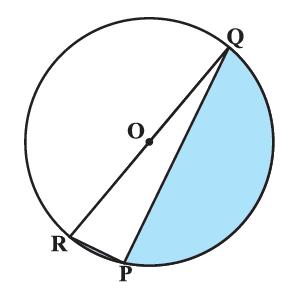# Ex.12.3 Q1 Areas Related to Circles Solution - NCERT Maths Class 10

## Question

Find the area of the shaded region in the figure, if $$PQ = 24\,\rm{cm},$$ $$PR = 7\rm\,{cm}$$ and $$O$$ is the centre of the circle.Video Solution
Areas Related To Circles
Ex 12.3 | Question 1

## Text Solution

What is known?

$$PQ = 24\, {\rm{cm}}, PR = 7\,{\rm{cm}},\, O$$ is the center the circle.

Use \begin{align}\pi = \frac{{22}}{7}\end{align}

What is unknown?

Area of the shaded region in figure.

Reasoning:

(i) Visually it’s clear that

Area of the shaded region $$=$$ Area of semicircle $$RPQ \,-$$  Areaof $$\Delta RPQ$$

\begin{align}= {\frac{1}{2}\pi = {{(OR)}^2} - {\text{Area of }}\Delta \rm{RPQ}}\end{align}

Since we don’t know $$RQ$$ (diameter) $$OR$$ and $$PQ$$ (radii of circle), we are unable to find either area of semicircle and we don’t know $$RQ$$ so we can’t find area of $$\Delta RPQ$$ using heron’s formula (since either $$2$$ sides are known) or by\begin{align} \frac{1}{2} \times {\text{base}} \times {\text{height}}\end{align} as we have only $$2$$ sides and that their respective heights.

So, we need to find $$RQ.$$

(ii) Using the knowledge:

Angle subtended by an arc at any point on the circle is half of the angle subtended by it at the center.

\begin{align} \therefore \angle ROQ &= {{180}^\circ } \\ \therefore \angle RPQ &= \frac{1}{2} \times {{180}^\circ = {{90}^\circ }} \end{align}

($$\rm{OR}$$) angle in a semicircle $$= {90^ \circ }$$

We get $$\Delta RQP$$ is a right angle triangle.

Using Pythagoras theorem

\begin{align} RQ^2 &= PR^2 + PQ^2 \end{align}

We get \begin{align} RQ &= \sqrt PR^2 + {PQ^2} \\\therefore \text{radius} &= \frac{1}{2}\,RQ.\end{align}

We can find the area of semicircle.

And since $$\angle RPQ = {90^ \circ }$$

$$\therefore\; RP$$ is the height for $$PQ$$ or vice versa

So using the formula.

Area of \begin{align} \Delta = \frac{1}{2} \times {\text{base}} \times {\text{height}}\end{align}

Area of \begin{align} \Delta \,RPQ = \frac{1}{2} \times PQ \times RP \end{align}

Steps:

$$PQ = 24\,{\rm{cm}}\,\,, PR = 7\,\rm{cm}$$

Hence the angle in a semicircle is a right angle

$$\therefore \;LRPQ = {90^\circ }$$

$$\Rightarrow \Delta {RQP}$$ is a right angled triangle.

$$\therefore \;$$ Using Pythagoras theorem.

\begin{align}{P{Q^2}} &= {P{R^2}} + {P{Q^2}}\\{RQ }&= \sqrt {{7^2} + {{24}^2}} \\ &= \sqrt {49 + 576} \\ &= \sqrt {625} \\ &= 25\,{\rm{cm}}\end{align}

$$\therefore\;$$Radius ($$r$$)  \begin{align}= \frac{{25}}{2}{\text{cm}}\end{align}

Area of shaded region$$=$$Area of semicircle $$RPQ\; –$$ Area of $$\Delta RQP$$

\begin{align}&= \frac{1}{2} \times \pi {{\text{r}}^{\text{2}}} - \frac{{\text{1}}}{{\text{2}}} \times {{PQ}} \times {RP}\\ &= \frac{1}{2} \times \left[ {\frac{{22}}{7} \times \frac{{25}}{2} \times \frac{{25}}{2} - 24 \times 7} \right]\\ &= \frac{1}{2} \times \left[ {\frac{{6875}}{{14}} - 168} \right]\\ &= \frac{1}{2} \times \left[ {\frac{{6875 - 2352}}{{14}}} \right] = \frac{1}{2} \times \frac{{4523}}{{14}}\\ &= \frac{{4523}}{{28}}\,{\text{cm}^2}\\& = 161.535\,\,{\text{cm}^2}\\ &= 161.54\,\,{\text{cm}^2}\,\left( {{\text{approximately}}} \right)\end{align}

Learn from the best math teachers and top your exams

• Live one on one classroom and doubt clearing
• Practice worksheets in and after class for conceptual clarity
• Personalized curriculum to keep up with school